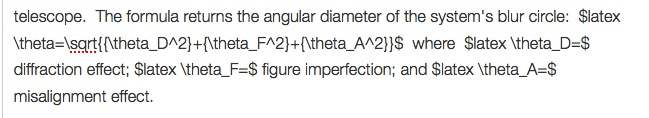# Why collimate? And an excuse to try LaTeX

I’ve been enjoying a book i got on sale thru SPIE, Friedman and Miller’s “Photonics Rules of Thumb”.  Much of it is (literally) rocket science, but there are wonderful offerings for the advanced beginner, especially in the introductory sections.  One tidbit amateur astronomers may find interesting is a formula relating to the optical performance of a telescope.  The formula returns the angular diameter of the system’s blur circle:$\theta=\sqrt{{\theta_D^2}+{\theta_F^2}+{\theta_A^2}}$  where$\theta_D=$ diffraction effect;$\theta_F=$ figure imperfection; and$\theta_A=$ misalignment effect.  One immediately sees that an acceptable blur circle from diffraction and optical figure can be blown by poor alignment.  “Having an element with exceptional figure does not provide a smaller spot if the alignment can’t be done properly”

I also wanted an excuse to try out LaTeX in WordPress, using the built-in Jetpack dingus. It makes the math looks really great and obscures the fact that I’m somewhat math impaired. I found the site at http://web.ift.uib.no/Teori/KURS/WRK/TeX/symALL.html to be helpful, as was “The Short Math Guide to Latex”  at ftp://ftp.ams.org/ams/doc/amsmath/short-math-guide.pdf  I looked at many previews before publishing.  Here’s what the LaTex code looks like: